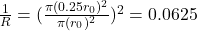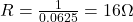## The resistance of a wire of lengt L0 and radius r0 is 1 ohm If the wire is stretched so thay its radius becomes 0.25r0. what is the new resi

Question

The resistance of a wire of lengt L0 and radius r0 is 1 ohm If the wire is stretched so thay its radius becomes 0.25r0. what is the new resistance of the wire

in progress 0
1 month 2021-09-05T16:08:25+00:00 1 Answers 0 views 0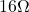Explanation:

Initial length of wire=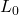Initial radius of wire=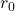Resistance of wire=1 ohm

After stretching

Radius of wire=r=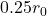New resistance  of wire=R

When the wire drawn under tensile stress  then volume remains constant.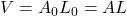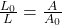…(1)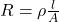Using the formula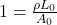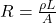Using equation (1)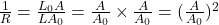Area , A=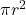Substitute the values